## June 12th, 2012

### Horizontal Perspective Correction in Text Images

I was recently working on a computer vision problem which I would like to share with you. We were dealing with images of a restaurant menu containing mostly text. Images were taken with a mobile phone camera while the user held the menu in his hand. Typically the image captures just part of the page (page boundaries were not necessarily included). The challenge was to perform perspective correction on such images. We treated this as a practical, rather than an academic problem and thus did not aim to get the perfect solution, so we were ready to accept one offering only a partial improvement.
The main, hopefully new, contributions of our approach are:
1. Partial, horizontal-only perspective correction.
2. A new, statistical approach of choosing a horizontal vanishing point.
The problem of perspective correction is well studied. Please see references at the end of this post for related work. The basic idea is as follows: Given a pair of parallel lines, we can find a vanishing point. In a non-distorted image, all vanishing points lie at the line at infinity. However, in a image projection, parallel lines may intersect at a real point. Given two pairs of lines which are supposed to be parallel (before projection), we can find two vanishing points which would define a line at infinity after perspective distortion. The idea is illustrated below. Vh and Vv are horizontal and vertical vanishing points respectively.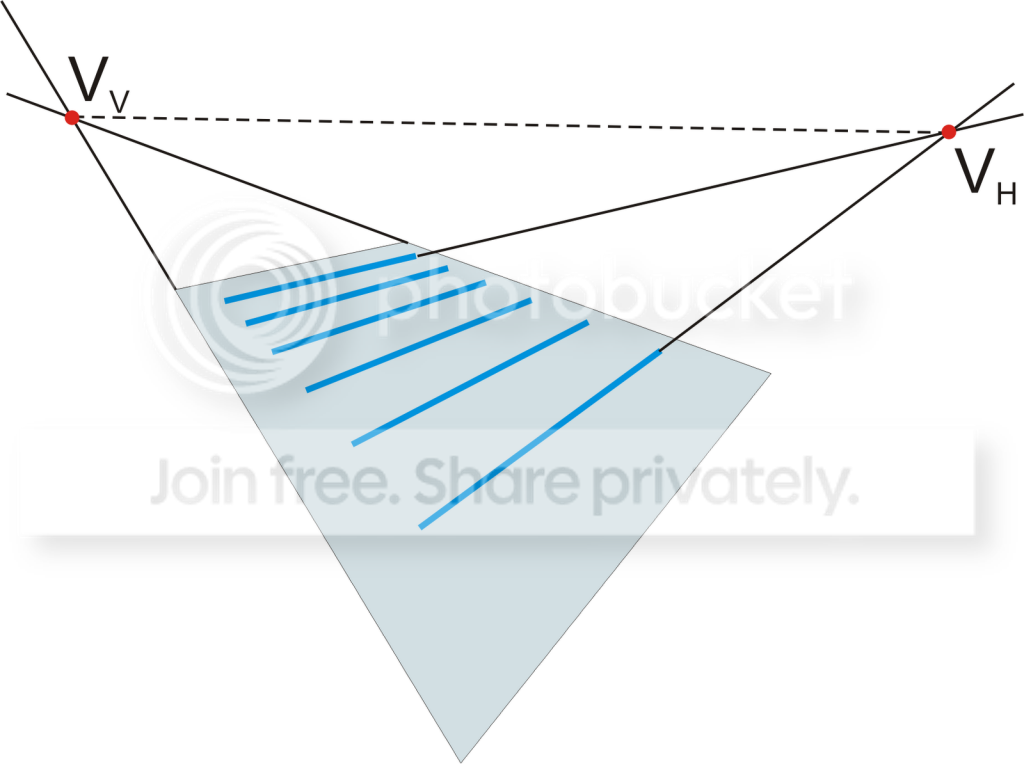Now, working in homogeneous coordinates , we can build a homography , which would translate this line to an ideal line at infinity. Applying this homography to all pixels of a perspective-distorted image would allow us to reconstruct the original.
Our first task is to find two pairs of line in the distorted imags which were parallel in the original image. One obvious idea is to find horizontal lines corresponding to lines of printed text. The intersection of two such lines would give us a horizontal vanishing point. For the type of images we are dealing with, unfortunately there is a no easy way to detect vertical lines. In absence of vertical page margins in typical images, there are simply not enough vertical features with which to align such lines. So we must resign ourselves to partial perspective correction, using the horizontal vanishing point alone. This is done by assuming that the vertical vanishing point is located at infinity in the positive direction of the y axis. The coordinate of such a point in homogeneous coordinates would be (0,1,0), with the last zero indicating an ideal point (point at infinity).

Starting with a pre-processed image (already converted to black and white, we will apply Hough transform  to detect straight lines. Because we are interested in horizontal lines, assuming that we are not dealing with extreme cases of images being significantly distorted, we can limit the line angles that we consider to +-Pi/3 from the x axis. The transformation could be applied to a scaled down image, provided that the aspect ratio is preserved. This would help us to speed up the computations. Next, we will threshold the results of the Hough transform and convert the resulting detected lines from polar to homogeneous coordinates. As a result, we will have a small set of potentially horizontal lines. In theory any pair of them should suffice, but in practice, some detected lines may not correspond to horizontal lines of text, but represent a noise or other image features.

To select the two most suitable lines from this set, we will use a statistical approach. First we will build a pairwise intersection of all lines from the set. In homogeneous coordinates, the intersection of two lines is a cross product of their coordinate vectors. This gives us a set of a potential horizontal vanishing points. We will filter this set excluding points at infinity, points falling within the bounding rectangle of the projected image, and to exclude extreme cases of perspective distortion, the points located too close to the center of coordinates in the horizontal direction. The “too close” criteria is expressed as a threshold on an absolute value of the  ratio  of the x coordinate of a potential horizontal vanishing point to the image width.  Each of the remaining points could be used to calculate a homography used to perform horizontal perspective correction:where (a,b,c) is a projection of the line at infinity calculated as the cross product of Vx and Vy=(0,1,0). As a result of such correction, the two lines used to produce a chosen point will become parallel. Our working assumption is that because of the regular text line structure of the original image, most of the lines we detected were parallel. This allows us to define an evaluation metric of suitability for a homography as the standard deviation of the angles between all corrected lines and the x axis. The vanishing point producing the minimal standard deviation will correspond to the transformation which makes the set of lines the closest to parallel.
The resulting homography performs perspective correction, making our set of original horizontal lines to become closer to parallel after transformation. However, this does not make them actually horizontal. We need an additional affine rotation homography to achieve this. After perspective transformation, we will take the mean value of angles between lines in our set and  the x axis as a rotation angle theta. The homography representing  this rotation would be: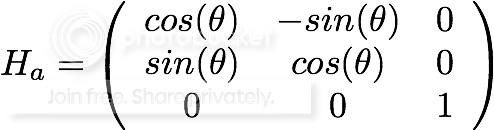The final homography combining perspective correction and rotation is the combination of the two transformations
H = Ha Hp

which coincidentally have the form: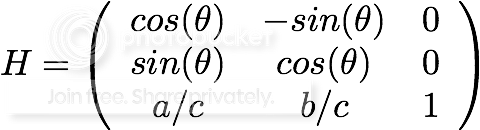Sample results of the algorithm are shown below. Lines used for horizontal correction are shown in orange. The original image: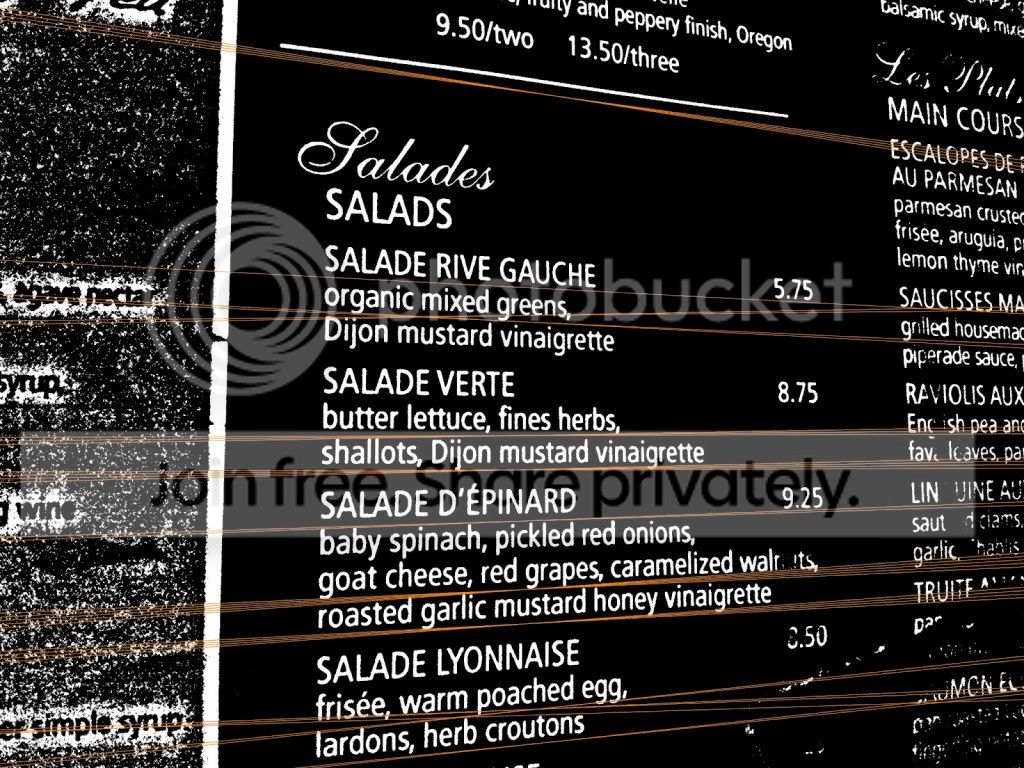The corrected image: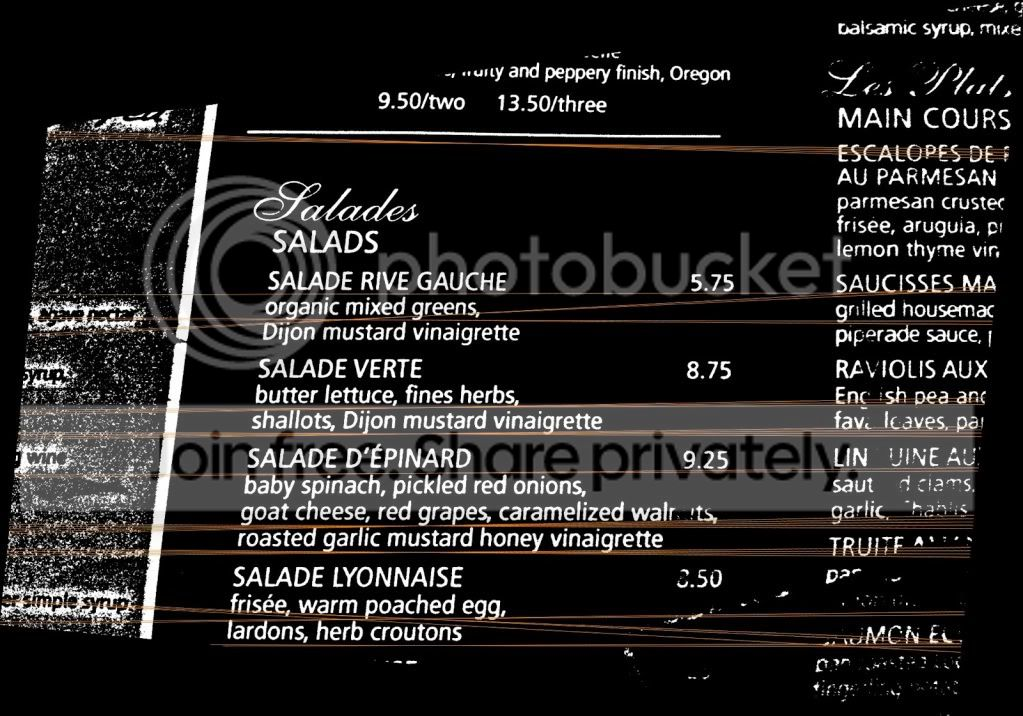References
 R. Hartley and A. Zisserman, "A multiple view geometry in computer vision", Second Edition, Cambridge University Press , 2004.
 P. Clark, “Estimating the orientation and recovery of text planes in a single image,” Proceedings of the 12th British Machine, 2001.
 V. Cantoni, L. Lombardi, and M. Porta, “Vanishing point detection: Representation analysis and new approaches,” Image Analysis and, no. Iciap, 2001.
 L. Jagannathan, “Perspective correction methods for camera based document analysis,” on Camera-based Document Analysis and, pp. 148-154, 2005.
 Wikipedia contributors. Homogeneous coordinates. Wikipedia, The Free Encyclopedia. May 22, 2012, 07:12 UTC. Available at: http://en.wikipedia.org/w/index.php?title=Homogeneous_coordinates&oldid=493787558. Accessed June 12, 2012.
 Wikipedia contributors. Hough transform. Wikipedia, The Free Encyclopedia. June 11, 2012, 00:12 UTC. Available at: http://en.wikipedia.org/w/index.php?title=Hough_transform&oldid=496983042. Accessed June 12, 2012.
 Wikipedia contributors. Homography. Wikipedia, The Free Encyclopedia. March 12, 2012, 06:33 UTC. Available at: http://en.wikipedia.org/w/index.php?title=Homography&oldid=481473030. Accessed June 12, 2012.